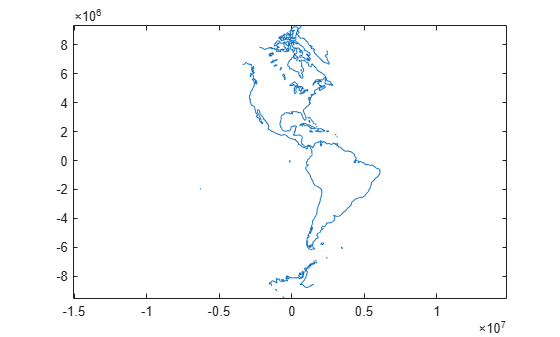# Project Coordinates Without Map Axes

This example shows how to perform the same projection computations that are done within Mapping Toolbox display commands by calling the `defaultm` and `mfwdtran` functions.

Create an empty map projection structure for a Sinusoidal projection, using the `defaultm` function. The function returns an `mstruct` .

`mstruct = defaultm('sinusoid');`

Set the map limits for the `mstruct` . To populate the fields of the map projection structure and ensure the effects of property settings are properly implemented, call `defaultm` a second time. Note that the longitude of the origin is centered between the longitude limits.

```mstruct.maplonlimit = [-150 -30]; mstruct.geoid = referenceEllipsoid('grs80','kilometers'); mstruct = defaultm(mstruct); mstruct.origin```
```ans = 1×3 0 -90 0 ```

Trim the coast to the map limits.

```load coastlines [latt,lont] = maptriml(coastlat,coastlon, ... mstruct.maplatlimit,mstruct.maplonlimit);```

Project the latitude and longitude vectors into plane coordinates with the Sinusoidal projection and display the result using standard MATLAB graphics commands. The resulting plot shows that data are projected in the specified aspect.

```[x,y] = mfwdtran(mstruct,latt,lont); figure plot(x,y) axis equal```##### SupportGet trial now